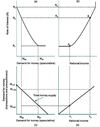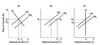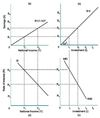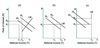Module 19 - Real and Monetary Sectors Flashcards Preview

Economics > Module 19 - Real and Monetary Sectors > Flashcards

Flashcards in Module 19 - Real and Monetary Sectors Deck (41):
1

2

3

4

Which of the following correctly states what the speculative demand for money would be if the level of national income lay between Y1 and Y2? It would be A. greater than MT + P1 but less than MT + P2. B. greater than MS2 but less than MS1 C. greater than 0 but less than MS2 D. greater than 0 but less than MT + P15

If the transaction demand for money were to increase at each and every level of income this would have the effect of I. shifting the money supply curve to the right II. shifting the speculative demand for money to the left III. shifting the LM curve to the left Which of the following is correct? A. I only B. II and III only C. III only D. I, II and III6

The horizontal portion of the LM curve would extend rightwards beyond point A if I. the transaction demand for money decreased II. the money supply decreased Which of the following is correct? A. I only B. II only C. Both I and II D. Neither I nor II7

If real world numbers for a given country were inserted on the vertical and horizontal axes of the LM diagram what would the limits of those numbers be I. 4% for the real rate of interest, the maximum growth rate of potential output. II. potential national output. Which of the following is correct? A. I only B. II only C. Both I and II D. Neither I nor II8

The LM curve would shift from LM2 to LM1 if I. the money supply increased II. the rate of interest increased Which of the following is correct? A. I only B. II only C. Both I and II D. Neither I nor II9

In Figure (c) the shift in the LM curve from LM1 to LM2 did not cause Y to increase. This was because I. the economy was at full employment II. the shift was not caused by an increased in the money supply III. investment expenditure is interest rate inelastic Which of the following is correct? A. I only B. I and II only C. III only D. I, II and III10

In Figure (a) the increase in the money supply shifts the LM curve to the right causing R to decrease from R1 to R2 which in turn causes I. investment expenditure to increase II. consumption expenditure to increase. both of which lead to Y increasing from Y1 to Y2 Which of the following is correct? A. I only B. II only C. Both I and II D. Neither I nor II11

12

13

14

Which of the following identifies correctly what the equilibrium rate of interest would be if the level of national income lay between Y1 and Y2? It would be A. greater than zero but less than S1 B. greater than S1 but less than S2 C. greater than R1 but less than R2 D. greater than R2 but less than R315

If the consumption function shifted so that the savings function remained linear arising in the origin but passed through the point S1Y2 then the I. S = I schedule would shift upwards II. MEI schedule would shift to the right III. IS curve would shift to the right Which of the following is correct? A. I and II only B. III only C. I, II and III D. Not I, not II, not III16

If businesses become pessimistic about the future in light of rising unemployment and postponed investment expenditures they were about to make this would cause I. the MEI schedule to shift to the right II. the savings function to shift downwards III. the IS curve to shift to the right Which of the following is correct? A. I only B. II only C. I and III only D. Not I, not II, not III17

If an international sector with a balance of trade surplus were to be added to the figure, which of the following would be true? A. The S = I line would no longer be a 45° line B. The S = I line would no longer pass through the origin C. The MEI schedule would shift to the right D. The savings function would have a different slope18

The shift in the IS curve from IS1 to IS2 could have resulted from I. an increase in government expenditure II. an increase in the income tax rate III. an increase in exports Which of the following is correct? A. I and III only B. II only C. I, II and III D. Not I, not II, not III19

Which of the following identifies the diagram in which a given cut in the tax rate would have the largest impact on national income and explains why? A. Diagram (a) because the increase in Y is equal approximately to the increase in R B. Diagram (b) because there is no negative monetary/interest rate multiplier C. Diagram (c) because the economy has reached full employment D. Diagram (b) because the increase in demand is matched by an increase in the money supply thus keeping R constant20

Crowding out is in evidence in the figure. When IS shifts because of an increase in government expenditure I. there is partial crowding out in (a) II. there is complete crowding out in (b) III. there is complete crowding out in (c) Which of the following is correct? A. I only B. II only C. I and II only D. I and III only21

22

23

24

25

Which of the following is correct in relation to Figure 19.15? A. To achieve full employment using only monetary policy, the money supply would have to be increased by the amount BC. B. To achieve full employment using only monetary policy, the money supply would have to be increased by the amount AC. C. To achieve full employment using only monetary policy, the money supply would have to be decreased by an amount BC − AB. D. It is not possible to achieve full employment just by increasing the money supply.26

Which of the following statements is correct with respect to point D in Figure 19.15? A. Point D cannot be reached because it implies a level of national income greater than full-employment national income. B. Point D can be achieved, but it would produce an inflationary gap. C. Point D can be achieved by a combination of tax cuts and increases in government expenditure with the money supply constant. D. Point D can produce equilibrium in both the money and real goods markets by an increase in the money supply with no changes in fiscal policy.27

On the basis of Figure 19.15, the government desires to achieve full employment YF, to raise the rate of interest to R3, and to have equilibrium in both the real goods and money markets. Which of the following policies could achieve all of these goals? A. An increase in government expenditure and increase in the money supply. B. A decrease in taxes and a decrease in the money supply. C. An increase in government expenditure and decrease in taxes. D. A decrease in the money supply.28

29

30

31

32

33

34

35

36

37

38

39

40

41